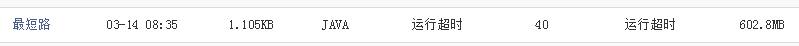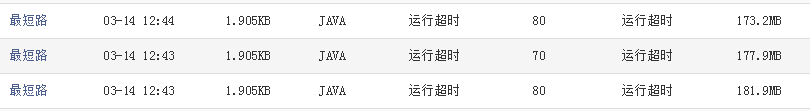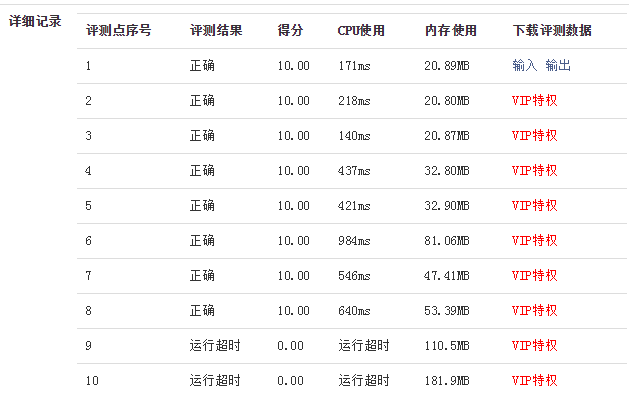• 蓝桥杯 最短路
                问题描述给定一个n个顶点，m条边的有向图（其中某些边权可能为负，但保证没有负环）。请你计算从1号点到其他点的最短路（顶点从1到n编号）。输入格式第一行两个整数n, m。接下来的m行，每行有三个整数u, v, l，表示u到v有一条长度为l的边。输出格式共n-1行，第i行表示1号点到i+1号点的最短路。 样例输入3 31 2 -12 3 -13 1 2 样例输出-1-2 数据规模与约定对于10%的数据，n = 2，m = 2。对于30%的数据，n <= 5，m <= 10。对于100%的数据，1 <= n <= 20000，1 <= m <= 200000，-10000 <= l <= 10000，保证从任意顶点都能到达其他所有顶点。 标准的SPFA模板题。。。。 #include <stdio.h>#include <string.h>#include <algorithm>#include <queue>using namespace std;const int inf = 1<<30;const int L = 200000;struct Edges{    int x,y,w,next;} e[L<<2];int head[L];int dis[L];int vis[L];int cnt[L];void AddEdge(int x,int y,int w,int k){    e[k].x = x,e[k].y = y,e[k].w = w,e[k].next = head[x],head[x] = k;}int relax(int u,int v,int c){    if(dis[v]>dis[u]+c)    {        dis[v] = dis[u]+c;        return 1;    }    return 0;}int SPFA(int src){    int i;    memset(cnt,0,sizeof(cnt));    dis[src] = 0;    queue<int> Q;    Q.push(src);    vis[src] = 1;    cnt[src]++;    while(!Q.empty())    {        int u,v;        u = Q.front();        Q.pop();        vis[u] = 0;        for(i = head[u]; i!=-1; i=e[i].next)        {            v = e[i].y;            if(relax(u,v,e[i].w)==1 && !vis[v])            {                Q.push(v);                vis[v] = 1;            }        }    }}int main(){    int t,n,m;    int i,j;    scanf("%d%d",&n,&m);    memset(e,-1,sizeof(e));    for(i = 1; i<=n; i++)    {        dis[i] = inf;        vis[i] = 0;        head[i] = -1;    }    for(i = 0; i<m; i++)    {        int x,y,w;        scanf("%d%d%d",&x,&y,&w);        AddEdge(x,y,w,i);    }    SPFA(1);    for(i = 2; i<=n; i++)        printf("%d\n",dis[i]);    return 0;}


展开全文• 请你计算从1号点到其他点的最短路(顶点从1到n编号)。输入格式第一行两个整数n, m。接下来的m行，每行有三个整数u, v, l，表示u到v有一条长度为l的边。输出格式共n-1行，第i行表示1号点到i+1号点的最短路。样例输入3 ...
问题描述给定一个n个顶点，m条边的有向图(其中某些边权可能为负，但保证没有负环)。请你计算从1号点到其他点的最短路(顶点从1到n编号)。输入格式第一行两个整数n, m。接下来的m行，每行有三个整数u, v, l，表示u到v有一条长度为l的边。输出格式共n-1行，第i行表示1号点到i+1号点的最短路。样例输入3 31 2 -12 3 -13 1 2样例输出-1-2数据规模与约定对于10%的数据，n = 2，m = 2。对于30%的数据，n <= 5，m <= 10。对于100%的数据，1 <= n <= 20000，1 <= m <= 200000，-10000 <= l <= 10000，保证从任意顶点都能到达其他所有顶点。import java.io.BufferedReader;import java.io.IOException;import java.io.InputStreamReader;import java.io.OutputStreamWriter;import java.io.PrintWriter;import java.io.StreamTokenizer;import java.util.ArrayDeque;import java.util.ArrayList;import java.util.List;import java.util.Queue;public class zuiduanlu {static int leng[];public static void main(String[] args) throws IOException {// TODO 自动生成的方法存根StreamTokenizer in=new StreamTokenizer(new BufferedReader(new InputStreamReader(System.in)));PrintWriter out = new PrintWriter(new OutputStreamWriter(System.out));in.nextToken();int n=(int)in.nval;in.nextToken();int m=(int)in.nval;Listlist[]=new ArrayList[n];//存储路径for(int i=0;i{list[i]=new ArrayList();}leng=new int[n];boolean jud[]=new boolean[n];//判断是否在队列内for(int i=1;ifor(int i=0;i{in.nextToken();int u=(int)in.nval;in.nextToken();int v=(int)in.nval;in.nextToken();int l=(int)in.nval;list[u-1].add(new node(v-1, l));}Queueq1=new ArrayDeque();q1.add(0);//第一个while(!q1.isEmpty()){int x=q1.poll();jud[x]=false;for(int i=0;i{int index=list[x].get(i).x;//x邻居该节点的编号int length=list[x].get(i).leng;//x到这个邻居的距离if(leng[index]>leng[x]+length){leng[index]=leng[x]+length;if(!jud[index])//队列中没有该点{q1.add(index);jud[index]=true;}}}}for(int i=1;i{out.println(leng[i]);}out.flush();}static class node{int x;int leng;public node(int x,int leng){this.x=x;this.leng=leng;}}}
展开全文• 请你计算从1号点到其他点的最短路(顶点从1到n编号)。输入格式第一行两个整数n, m。接下来的m行，每行有三个整数u, v, l，表示u到v有一条长度为l的边。输出格式共n-1行，第i行表示1号点到i+1号点的最短路。样例输入3 ...
1 问题描述问题描述给定一个n个顶点，m条边的有向图(其中某些边权可能为负，但保证没有负环)。请你计算从1号点到其他点的最短路(顶点从1到n编号)。输入格式第一行两个整数n, m。接下来的m行，每行有三个整数u, v, l，表示u到v有一条长度为l的边。输出格式共n-1行，第i行表示1号点到i+1号点的最短路。样例输入3 31 2 -12 3 -13 1 2样例输出-1-2数据规模与约定对于10%的数据，n = 2，m = 2。对于30%的数据，n <= 5，m <= 10。对于100%的数据，1 <= n <= 20000，1 <= m <= 200000，-10000 <= l <= 10000，保证从任意顶点都能到达其他所有顶点。2 解决方案2.1 floyd算法解决使用floyd算法(ps:算法笔记_069:Floyd算法简单介绍(Java))求解本题的时间复杂度为O(n^3)，下面代码在系统中测评分为40，原因：运行超时。以下代码仅供参考。具体代码如下：import java.util.Scanner;public class Main {public void floyd(long[][] adjMatrix) {for(int k = 0;k < adjMatrix.length;k++) {for(int i = 0;i < adjMatrix.length;i++) {for(int j  = 0;j < adjMatrix.length;j++) {if(adjMatrix[i][k] != Integer.MAX_VALUE && adjMatrix[k][j] != Integer.MAX_VALUE) {if(adjMatrix[i][j] > adjMatrix[i][k] + adjMatrix[k][j])adjMatrix[i][j] = adjMatrix[i][k] + adjMatrix[k][j];}}}}for(int i = 1;i < adjMatrix.length;i++)System.out.println(adjMatrix[i]);}public static void main(String[] args) {Main test = new Main();Scanner in = new Scanner(System.in);int n = in.nextInt();int m = in.nextInt();if(n > 20000 || n < 1 || m > 200000 || m < 1)return;long[][] adjMatrix = new long[n][n];for(int i = 0;i < n;i++) {for(int j = 0;j < n;j++)adjMatrix[i][j] = Integer.MAX_VALUE;}for(int i = 0;i < m;i++) {int a = in.nextInt();int b = in.nextInt();int value = in.nextInt();if(value > 10000 || value < -10000)return;adjMatrix[a - 1][b - 1] = value;}test.floyd(adjMatrix);}}2.2 spfa算法解决使用spfa算法(PS:算法笔记_071:SPFA算法简单介绍(Java))求解本题的时间复杂度为O(m*E)(PS:其中E为给定边的数目，m为图中顶点进出链表的总次数，一般不大于2*n，n为图中总顶点数)。下面的给出的代码，在系统中测评分为70或者80分(PS：同样代码提交了两次，评分不一样)，具体原因：运行超时。可能是Java语言编译时间没有C/C++那么快，所以不能在1s内完成。如果是楼主自己代码问题，还请路过同学不吝赐教啊~具体代码如下：import java.util.ArrayList;import java.util.LinkedList;import java.util.Scanner;public class Main {static class edge {public int a;  //边的起点public int b;  //边的终点public int value;  //边的权值edge(int a, int b, int value) {this.a = a;this.b = b;this.value = value;}}public void spfa(ArrayList[] listA, int n) {long[] result = new long[n];int[] num = new int[n];boolean[] used = new boolean[n];for(int i = 1;i < n;i++) {result[i] = Integer.MAX_VALUE;used[i] = false;}LinkedList list = new LinkedList();list.add(0);num = 1;used = true;while(list.size() > 0) {int start = list.getFirst();for(int i = 0, length = listA[start].size();i < length;i++) {int b = listA[start].get(i).b;int value = listA[start].get(i).value;if(result[b - 1] > result[start] + value) {result[b - 1] = result[start] + value;if(!used[b - 1]) {used[b - 1] = true;list.add(b - 1);num[b - 1]++;if(num[b - 1] > n)return;}}}list.removeFirst();used[start] = false;}for(int i = 1;i < n;i++)System.out.println(result[i]);return;}public static void main(String[] args) {Main test = new Main();Scanner in = new Scanner(System.in);int n = in.nextInt();int m = in.nextInt();if(n > 20000 || n < 1 || m > 200000 || m < 1)return;@SuppressWarnings("unchecked")ArrayList[] listA = new ArrayList[n];for(int i = 0;i < n;i++)listA[i] = new ArrayList();for(int i = 0;i < m;i++) {int a = in.nextInt();int b = in.nextInt();int value = in.nextInt();if(value > 10000 || value < -10000)return;listA[a - 1].add(new edge(a, b, value));}test.spfa(listA, n);}}算法笔记&lowbar;052&colon;蓝桥杯练习Multithreading(Java)目录 1 问题描述 2 解决方案   1 问题描述 问题描述 现有如下一个算法: repeat ni times yi := y y := yi+1 end repeat 令n为你需要算加法的第 ...算法笔记&lowbar;083&colon;蓝桥杯练习 合并石子(Java)目录 1 问题描述 2 解决方案   1 问题描述 问题描述 在一条直线上有n堆石子,每堆有一定的数量,每次可以将两堆相邻的石子合并,合并后放在两堆的中间位置,合并的费用为两堆石子的总数.求把所有石子 ...算法笔记&lowbar;107&colon;蓝桥杯练习  算法提高 学霸的迷宫(Java)目录 1 问题描述 2 解决方案   1 问题描述 问题描述 学霸抢走了大家的作业,班长为了帮同学们找回作业,决定去找学霸决斗.但学霸为了不要别人打扰,住在一个城堡里,城堡外面是一个二维的格子迷宫,要 ...算法笔记&lowbar;096&colon;蓝桥杯练习 算法提高 求最大值(Java)目录 1 问题描述 2 解决方案   1 问题描述 问题描述 给n个有序整数对ai bi,你需要选择一些整数对 使得所有你选定的数的ai+bi的和最大.并且要求你选定的数对的ai之和非负,bi之和非负 ...算法笔记&lowbar;091&colon;蓝桥杯练习 递推求值(Java)目录 1 问题描述 2 解决方案   1 问题描述 问题描述 已知递推公式: F(n, 1)=F(n-1, 2) + 2F(n-3, 1) + 5, F(n, 2)=F(n-1, 1) + 3F(n- ...算法笔记&lowbar;056&colon;蓝桥杯练习 未名湖边的烦恼(Java)目录 1 问题描述 2 解决方案 2.1 递归法 2.2 递推法   1 问题描述 问题描述 每年冬天,北大未名湖上都是滑冰的好地方.北大体育组准备了许多冰鞋,可是人太多了,每天下午收工后,常常一双冰 ...算法笔记&lowbar;055&colon;蓝桥杯练习 Tricky and Clever Password (Java)目录 1 问题描述 2 解决方案   1 问题描述 问题描述 在年轻的时候,我们故事中的英雄——国王 Copa——他的私人数据并不是完全安全地隐蔽.对他来说是,这不可接受的.因此,他发明了一种密码,好 ...算法笔记&lowbar;076&colon;蓝桥杯练习 结点选择(Java)目录 1 问题描述 2 解决方案   1 问题描述 问题描述 有一棵 n 个节点的树,树上每个节点都有一个正整数权值.如果一个点被选择了,那么在树上和它相邻的点都不能被选择.求选出的点的权值和最大是多 ...算法笔记&lowbar;060&colon;蓝桥杯练习 出现次数最多的整数(Java)目录 1 问题描述 2 解决方案   1 问题描述 问题描述 编写一个程序,读入一组整数,这组整数是按照从小到大的顺序排列的,它们的个数N也是由用户输入的,最多不会超过20.然后程序将对这个数组进行统 ...随机推荐基于select的python聊天室程序python网络编程具体参考. 在python中,select函数是一个对底层操作系统的直接访问的接口.它用来监控sockets.files和 ...Mongoose简单的连表查询原文摘自我的前端博客,欢迎大家来访问 http://www.hacke2.cn 像我这篇文章所说的基于Node.js + jade + Mongoose 模仿gokk.tv,当时停止开发是因为我深深的 ...nginx 日志分割利用 crontab + shell 来实现nginx的 access log 按天切割,便于统计.具体实现如下: shell: #! /bin/sh NGINX_DIR=/data/apps/ngi ...linux学习笔记2-命令总结4帮助命令 help - 帮助命令 man - 获取帮助信息 用户管理命令 useradd - 添加新用户 passwd - 设置用户密码 who - 显示所有用户 w - 查看更详细的用户信息 use ...CSS中如何将li横向排列直接贴段例子代码吧: @{ Layout = null;} 发布Activex全过程C#制作.打包.签名.发布Activex全过程 一.前言 最近有这样一个需求,需要在网页上面启动客户端的软件,软件之间的通信.调用,单单依靠HTML是无法实现了,因此必须借用Activex来实现.由于 ...Lightoj 1004 - Monkey Banana Problem题目链接:http://acm.hust.edu.cn/vjudge/contest/121396#problem/F http://lightoj.com/volume_showproblem.ph ...python之集合及其方法---整理集集合的定义: 由不同元素组成.一组无序排列的可hash值.集合中元素必须是不可变类型 集合的定义方式: 由大括号组成: 每个元素用逗号分隔: 元素书写不是key-value形式: 集合是由不同元素组成 ...shell怎么判断两个文件内容是否相同#cat diff_two_file#/bin/sbinfile1=/mnt/mmc/test/aafile2=/mnt/mmc/test/bbdiff $file1$file2 > /dev ...《linux系统及其编程》实验课记录(四)实验4:组织目录和文件 实验目标: 熟悉几个基本的操作系统文件和目录的命令的功能.语法和用法, 整理出一个更有条理的主目录,每个文件都位于恰当的子目录. 实验背景: 你的主目录中已经积压了一些文件,你 ...
展开全文• ## Java实现蓝桥杯最短路

万次阅读 多人点赞 2019-05-31 16:35:31
请你计算从1号点到其他点的最短路（顶点从1到n编号）。 输入格式 第一行两个整数n, m。 接下来的m行，每行有三个整数u, v, l，表示u到v有一条长度为l的边。 输出格式 共n-1行，第i行表示1号点到i+1号点的最短路...
问题描述
给定一个n个顶点，m条边的有向图（其中某些边权可能为负，但保证没有负环）。请你计算从1号点到其他点的最短路（顶点从1到n编号）。
输入格式
第一行两个整数n, m。
接下来的m行，每行有三个整数u, v, l，表示u到v有一条长度为l的边。
输出格式
共n-1行，第i行表示1号点到i+1号点的最短路。
样例输入
3 3
1 2 -1
2 3 -1
3 1 2
样例输出
-1
-2
数据规模与约定
对于10%的数据，n = 2，m = 2。
对于30%的数据，n <= 5，m <= 10。
对于100%的数据，1 <= n <= 20000，1 <= m <= 200000，-10000 <= l <= 10000，保证从任意顶点都能到达其他所有顶点。
import java.io.BufferedReader;
import java.io.IOException;
import java.io.OutputStreamWriter;
import java.io.PrintWriter;
import java.io.StreamTokenizer;
import java.util.ArrayDeque;
import java.util.ArrayList;
import java.util.List;
import java.util.Queue;

public class zuiduanlu {
static int leng[];
public static void main(String[] args) throws IOException {
// TODO 自动生成的方法存根
PrintWriter out = new PrintWriter(new OutputStreamWriter(System.out));
in.nextToken();
int n=(int)in.nval;in.nextToken();int m=(int)in.nval;
List<node>list[]=new ArrayList[n];//存储路径
for(int i=0;i<n;i++)//声明
{
list[i]=new ArrayList<node>();
}
leng=new int[n];
boolean jud[]=new boolean[n];//判断是否在队列内
for(int i=1;i<n;i++) {leng[i]=Integer.MAX_VALUE;}//初始最长均为max
for(int i=0;i<m;i++)
{
in.nextToken();int u=(int)in.nval;
in.nextToken();int v=(int)in.nval;
in.nextToken();int l=(int)in.nval;
}
Queue<Integer>q1=new ArrayDeque<Integer>();
while(!q1.isEmpty())
{
int x=q1.poll();
jud[x]=false;
for(int i=0;i<list[x].size();i++)//遍历
{
int index=list[x].get(i).x;//x邻居该节点的编号
int length=list[x].get(i).leng;//x到这个邻居的距离
if(leng[index]>leng[x]+length)
{
leng[index]=leng[x]+length;
if(!jud[index])//队列中没有该点
}
}
}
for(int i=1;i<n;i++)
{
out.println(leng[i]);
}
out.flush();
}
static class node
{
int x;
int leng;
public node(int x,int leng)
{
this.x=x;
this.leng=leng;
}
}

}




展开全文Java
• 请你计算从1号点到其他点的最短路（顶点从1到n编号）。 输入格式 第一行两个整数n, m。 接下来的m行，每行有三个整数u, v, l，表示u到v有一条长度为l的边。 输出格式 共n-1行，第i行表示1号点到i+1号点的最短路...
• 请你计算从1号点到其他点的最短路(顶点从1到n编号)。输入格式第一行两个整数n, m。接下来的m行，每行有三个整数u, v, l，表示u到v有一条长度为l的边。输出格式共n-1行，第i行表示1号点到i+1号点的最短路。样例输入3 ...
• 请你计算从1号点到其他点的最短路（顶点从1到n编号）。 输入格式 第一行两个整数n, m。 接下来的m行，每行有三个整数u, v, l，表示u到v有一条长度为l的边。 输出格式 共n-1行，第i行表示1号点到i+1号点的最短路。 ...算法 数据结构 程序人生 经验分享
• 蓝桥杯 最短路 这题用dijkstra过不了，只能用spfa.裸的spfa。 算法训练 最短路 时间限制：1.0s 内存限制：256.0MB 问题描述 给定一个n个顶点，m条边的有向图（其中某些边权可能为负，但保证没有负环）。请你...
• 请你计算从1号点到其他点的最短路（顶点从1到n编号）。 输入格式 第一行两个整数n, m。 接下来的m行，每行有三个整数u, v, l，表示u到v有一条长度为l的边。 输出格式 共n-1行，第i行表示1号点到i+1号点的最短路。 ...
• //v为记录是否到达过，pre记录点i优解父亲节点是谁 int dis[ 20002 ]={ 0 }; //从1到达i的最短长度 const int inf= 0x3f3f3f ; int main(){ int tmp1,tmp2; node date; scanf ( "%d%d" ,&n,&m)...
• 算法训练 最短路 时间限制：1.0s 内存限制：256.0MB 提交此题 锦囊1 锦囊2 问题描述 给定一个n个顶点，m条边的有向图（其中某些边权可能为负，但保证没有负环）。请你计算从1号点到其他点的最短路（顶点从1到n...
• 使用最短路算法。 锦囊2 使用Dijkstra算法，此图的边数比点数的平方要少很多，因此应该使用带堆优化的Dijkstra。 问题描述 给定一个n个顶点，m条边的有向图（其中某些边权可能为负，但保证没有负环）。请...
• 最短路作为算法入门的基本问题之一，大一开始学的时候也是稀里糊涂的，现在博主在大三上学了算法分析后，对这几种算法有了新的理解与认识，于是总结如下，希望能对自己以后的复习还有刚开始入坑算法的萌新们一点帮助...java
•  算法训练 最短路 时间限制：1.0s 内存限制：256.0MB 锦囊1 锦囊2 锦囊3 问题描述 给定一个n个顶点，m条边的有向图（其中某些边权可能为负，但保证没有负环）。请你计算从1号点到其他点的最短路...Java 最短路径
• 算法训练 最短路时间限制：1.0s 内存限制：256.0MB问题描述给定一个n个顶点，m条边的有向图(其中某些边权可能为负，但保证没有负环)。请你计算从1号点到其他点的最短路(顶点从1到n编号)。输入格式第一行两个整数n, m......